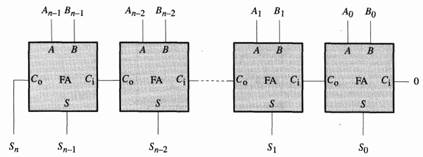Chat Now

# A common approach to the addition of n bit binary numbers uses n cascaded combinational modules each a l bit adder conventionally called a full adder Full adder modules can be combined as follows

### A common approach to the addition of n bit binary numbers uses n cascaded combinational modules each a l bit adder conventionally called a full adder Full adder modules can be combined as follows

A common approach to the addition of n,bit binary numbers uses n cascaded combinational modules, each a l-bit adder (conventionally called a full adder). Full-adder modules can be combined as follows to implement a circuit that adds the n-bit unsigned binary value A to the n-bit unsigned binary value B, yielding ~ the n-bit sum S (A0 designates the low-order bit of A, and A,-1 the high-order bit):A. What information is carried by the Ci input to each full adder? output?

B. Suppose the Ci input to the low-order full adder is connected to logical 1 rather than logical 0. Describe the relationship of the binary number S to the input numbers A and B under these circumstances.

C. Give truth tables for each of the outputs S and C, for the full-adder module in terms of its inputs A, B, and Ci. Draw Kamaugh maps for each output.

D. Draw a circuit diagram for a full-adder module, using AN, OR, NAND, XOR, and inverter modules. Try to minimize the number of component modules in your design. in; You’ll find an XOR gate useful.)

E. Assuming a tpd of 10 ns for each module, what propagation delay should be specified for the full adder? For the n-bit adder?

F. Propose an addition to the circuit to produce a single output bit U that carries a 1 if and only if the addition of the unsigned numbers A and B overflows the n-bit result S.

G. What modifications are necessary to make the n-bit adder properly add two signed numbers represented in 2’s complement binary? Explain.

H. Propose an addition to your (perhaps modified) circuit to produce an output V that carries a l if and only if the addition of the signed numbers A and B overflows the n-bit result S.

rahul 09-Jul-2020Get solution